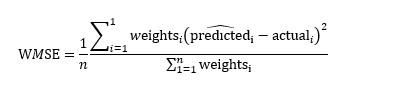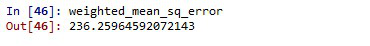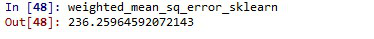Open in App
Not now

# How To Implement Weighted Mean Square Error in Python?

• Last Updated : 18 Mar, 2022

In this article, we discussed the implementation of weighted mean square error using python.

Mean squared error is a vital statistical concept, that is nowadays widely used in Machine learning and Deep learning algorithm. Mean squared error is basically a measure of the average squared difference between the estimated values and the actual value. It is also called a mean squared deviation and is most of the time used to calibrate the accuracy of the predicted output. In this article, let us discuss a variety of mean squared errors called weighted mean square errors.

Weighted mean square error enables to provide more importance or additional weightage for a particular set of points (points of interest) when compared to others. When handling imbalanced data, a weighted mean square error can be a vital performance metric. Python provides a wide variety of packages to implement mean squared and weighted mean square at one go, here we can make use of simple functions to implement weighted mean squared error.

### Formula to calculate the weighted mean square error:## Implementation of Weighted Mean Square Error

• For demonstration purposes let us create a sample data frame, with augmented actual and predicted values, as shown.
• Calculate the squared difference between actual and predicted values.
• Define the weights for each data point based on the importance
• Now, use the weights  to calculate the weighted mean square error as shown

Code Implementation:

## Python3

 `import` `pandas as pd ` `import` `numpy as np ` `import` `random ` ` `  `# create a dataset with actual and ` `# predicted values ` `d ``=` `{``'Actual'``: np.arange(``0``, ``20``, ``2``)``*``np.sin(``2``), ` `     ``'Predicted'``: np.arange(``0``, ``20``, ``2``)``*``np.cos(``2``)} ` ` `  `# convert the data to pandas dataframe ` `data ``=` `pd.DataFrame(data``=``d) ` ` `  `# create a weights array based on  ` `# the importance ` `y_weights ``=` `np.arange(``2``, ``4``, ``0.2``) ` ` `  `# calculate the squared difference ` `diff ``=` `(data[``'Actual'``]``-``data[``'Predicted'``])``*``*``2` ` `  `# compute the weighted mean square error ` `weighted_mean_sq_error ``=` `np.``sum``(diff ``*` `y_weights) ``/` `np.``sum``(y_weights) `

Output:Weighted Mean Square Error

Let us cross verify the result with the result of the scikit-learn package. to verify the correctness,

Code:

## Python3

 `# compare the results with sklearn package ` `weighted_mean_sq_error_sklearn ``=` `np.average( ` `    ``(data[``'Actual'``]``-``data[``'Predicted'``])``*``*``2``, axis``=``0``, weights``=``y_weights) ` ` `  `weighted_mean_sq_error_sklearn `

Output:verify the result

My Personal Notes arrow_drop_up
Related Articles